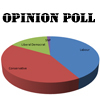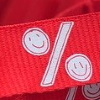#### You may also like### Mathland Election

A political commentator summed up an election result. Given that there were just four candidates and that the figures quoted were exact find the number of votes polled for each candidate.### Matching Fractions, Decimals and Percentages

Can you match pairs of fractions, decimals and percentages, and beat your previous scores?### Fractions and Percentages Card Game

Can you find the pairs that represent the same amount of money?

# Put Out the Flags

### Why do this problem?

This problem gives practice in calculating with percentages. It can be approached by trial and improvement but the equivalence between fractions and percentages is very useful.

### Key questions

What fraction is $50$%? and $35$%?
What would be a sensible number to try first?
Do you think they will not have a whole number of flags?
Tim could have $100$ flags but could he have less than $100$ flags?
If $50$% of Tim's flags are blue, what does this tell you about the number of flags Tim must have?
As $35$% of Tim'??s flags are red, what does this tell you about the number of flags Tim must have?

### Possible extension

Learners could devise and solve a second part to the question, similar to that one in the problem.

### Possible support

Suggest working with counters or drawing the flags starting with a suitable arbitrary number and working by trial and improvement.